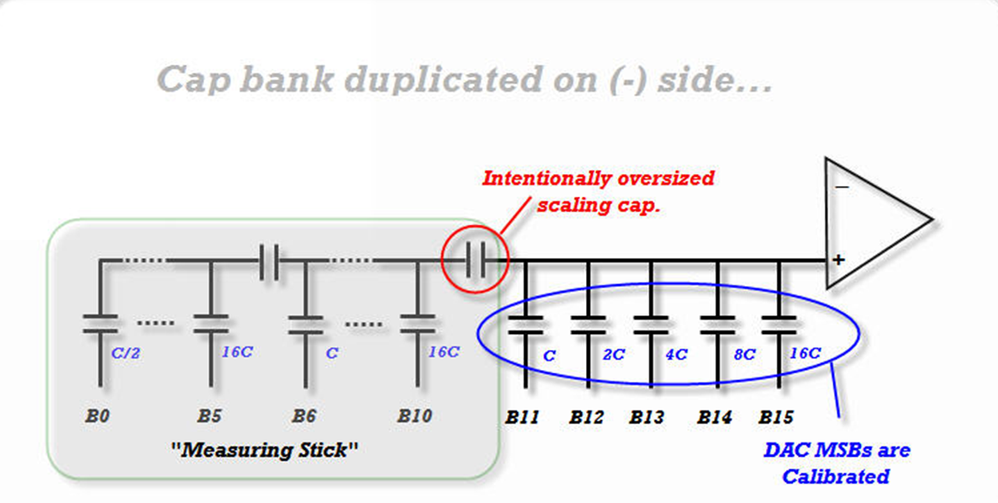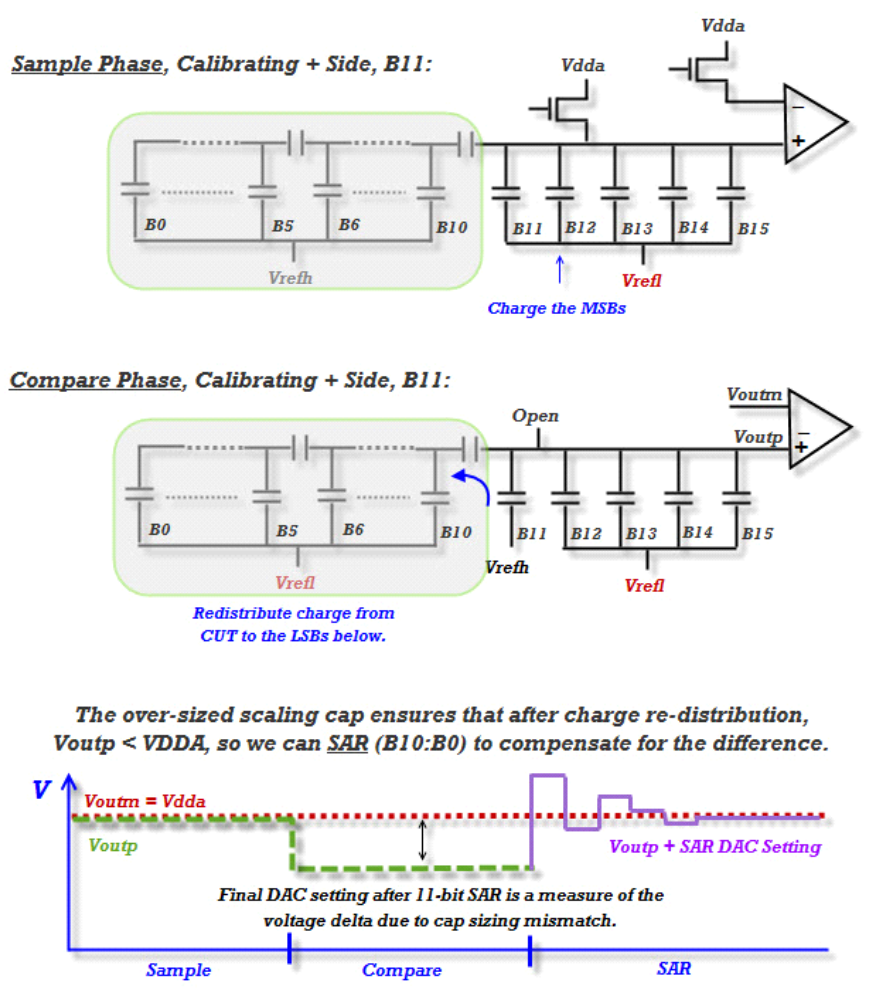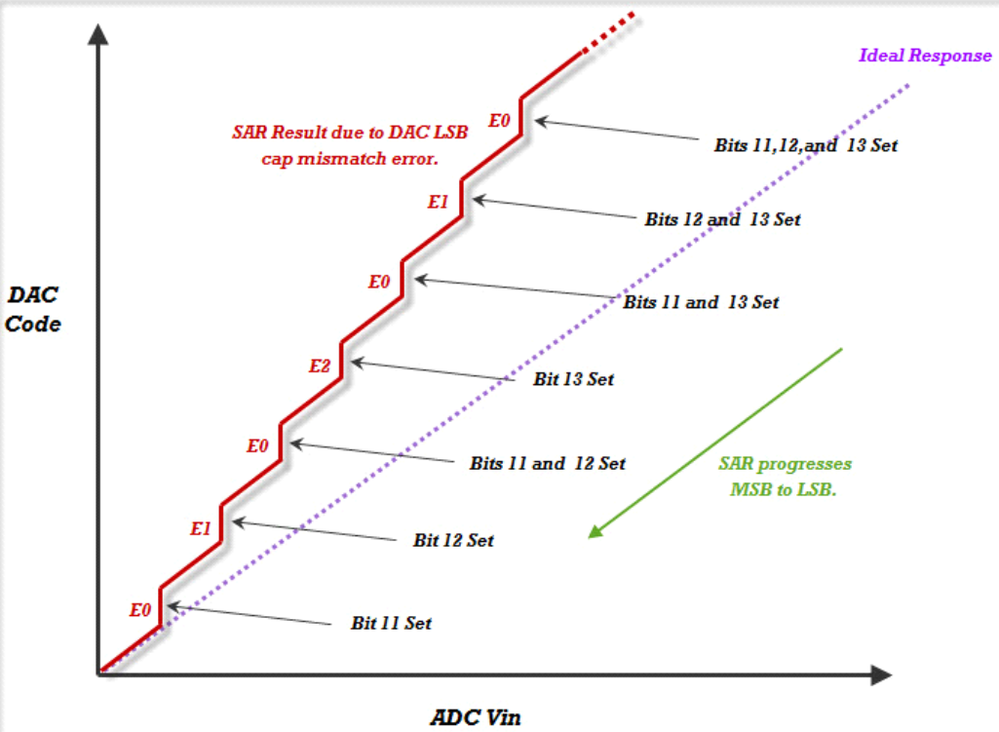cancel
Showing results for
Did you mean:

1. How Calibration works

There are three main sub-blocks important in understanding how the Kinetis SAR module works.  There is a capacitive DAC, a comparator, and the SAR engine that controls the module. Of those blocks, the

DAC is most susceptible to variations that can cause linearity problems in the SAR.

The DAC is architected with three sets of binary weighted capacitors arrayed in banks, as in Figure 1. The capacitors that represent the most significant bits of the SAR (B15:B11) are connected directly to the inputs of the comparator. The next bank of five capacitors (B10:B6) is connected to the top plate of the MSB array through an intentionally oversized scaling capacitor. The final six capacitors that makeup the least significant bits of the SAR (B5:B0) are correspondingly connected to the top plate of the middle bank of capacitors through another scaling capacitor.Figure 1. Arrangement of DAC capacitors

Only the MSB capacitor bank is calibrated. Because the first scaling capacitor is intentionally oversized, each of the non-calibrated MSB capacitors will have an effective capacitance too small to yield accurate results. However, because they are always too small, we can measure the amount oferror that each of those capacitors would cause individually, and add that back in to the result.

Calibration starts with the smallest of the LSB capacitors, B11. The SAR samples Vrefl on all of the capacitors that are lower-than or equal-to the capacitor under test (CUT), while connecting all of the smaller capacitors to Vrefh. The top plate of all of the MSB capacitors is held at VDDA while this happens. After the sampling phase is complete, the top plates of the MSB capacitors are allowed to float, and the bottom plates of the MSBs not under test are connected to Vrefl. This allows charge to redistribute from the CUT to the smaller capacitors. Finally, an 11 bit SAR algorithm (corresponding with the 11 capacitors that are smaller than the MSB array) is performed which produces a result that indicates the amount of error that the CUT has compared to an ideally sized capacitor. This process is repeated for each of the five MSBs on both the plus side and minus side DACs and the five error values that are reported correspond to the five MSBs accordingly. All of these error values are about the same magnitude, with a unit of 16-bit LSBs. See Figure 2 for an example.Figure 2. Example of calibration on bit 11

The DAC MSB error is cumulative. That is, if bit 11 of the DAC is set, then the error is simply the error of that bit. However if bit 12 of the DAC is set, the total error is equivalent tothe error reported on bit 12, plus the error reported on bit 11. For each MSB the error is calculated as below, where Ex is the error found during the calibration for its corresponding MSB bit:

• When bit 11 of the DAC is set: CLx0 = E0.
• When bit 12 of the DAC is set: CLx1 = E0+E1.
• When bit 13 of the DAC is set: CLx2 = E2 + E1 + 2E0.
• When bit 14 of the DAC is set: CLx3 = E3 + E2 + 2E1 + 4E0.
• When bit 15 of the DAC is set: CLx4 = E4 + 2E3 + 4E2 + 8E1 + 16E0Figure 3. Effect of calibration error on ADC response

These are the values that are then placed in each of the CLxx calibration results registers. Figure 3 shows how the errors would accumulate if all of the CLxx registers were set to zero. The offset and gain registers are calculated based on these values as well. Because of this, the gain and offset registers calibrate only for errors internal to the SAR itself. Self calibration does not compensate for board or system level gain or offset issues.

2. Recommended Calibration Procedure

From the above description it is evident that the calibration procedure is in effect several consecutive analog to digital conversions. These are susceptible to all of the same sources of error of any ADC conversion. Because what is primarily being measured is the error in the size of the MSB capacitors; the recommendation is to configure the SAR in such a way as to make for the most accurate conversions possible in the environment that the SAR is being calibrated in. Noise is the primary cause of run-to-run variation in this process,so steps should be taken to reduce the impact of noise during the calibration process. Such as:

• All digital IO should be silent and unnecessary modules should be disabled.
• The Vrefh should be as stable and high a voltage as possible, since higher Vrefh means larger ADC code widths. An isolated Vrefh pin would be ideal. Lacking that, using an isolated VDDA as the reference would be preferable to using VREFO.
• The clock used should be as noise free as possible, and less than or equal to 6 MHz. For this purpose the order of desirable clock sources for calibration would be OSC > PLL > FLL > ASYNC
• The hardware averaging should be set to the maximum 32 samples.
• The Low Power Conversion bit should be set to 0.
• The calibration should be done at room temperature.

The High Speed Conversion and Sample Time Adder will not have much effect in most situations, and the Diff and Mode bits are completely ignored by the calibration routine.

The calibration values should be taken for each instance of the SAR on a chip in the above conditions. They should be stored in nonvolatile memory and then written into their appropriate registers whenever the ADC register values are cleared.

In some instances, the system noise present will still cause the calibration routine to exhibit greater than desired run-to-run variation. One rule of thumb would be to repeat calibration several times and look at the CLx0 registers. If the value reported in that register varies by more than three, the following procedure can be implemented. Run the calibration routine several times. Twenty to forty times. Place the value of each of the calibration registers into a corresponding array. Perform a bubble sort on each array and find the median value for each of the calibration registers. Use  these median values as described for typical calibration results.

Labels (6)

• ### Kinetis W Series MCUs

Version history
Revision #:
1 of 1
Last update:
‎08-20-2014 07:07 AM
Updated by: